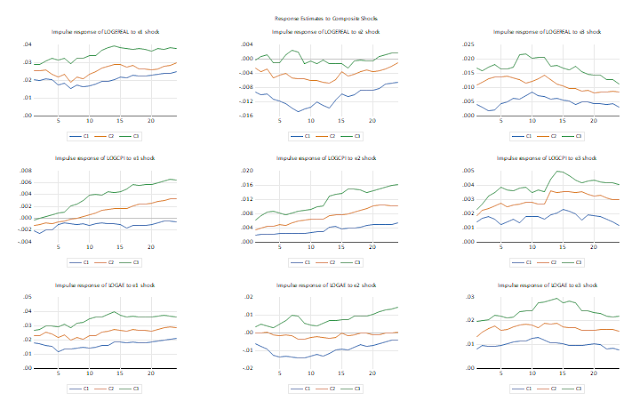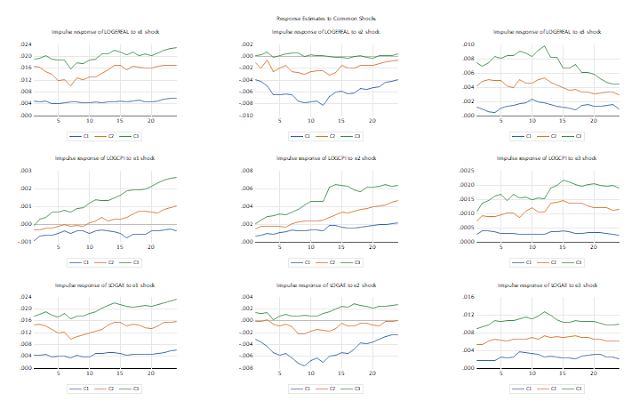## Tuesday, December 11, 2018

### Panel Structural VARs and the PSVAR add-in

Author and guest blog by Davaajargal Luvsannyam

Panel SVARs have been used to address a variety of issues of interest to policymakers and applied economists. Panel SVARs are particularly suitable to analyze the transmission of idiosyncratic shocks across units and time. For example, Canova et al. (2012) have studied how U.S. interest rate shocks are propagated to 10 European economies, 7 in the Euro area and 3 outside of it, and how German shocks are transmitted to the remaining nine economies.

Panel SVARs have also been often used to estimate average effects – possibly across heterogeneous groups of units - and to describe unit specific differences relative to the average. For example, researcher may analyze if monetary policy is more countercyclical, on average, in countries or states.  Researcher may also be interested in knowing whether inflation dynamics in states may depend on political, geographical, cultural or institutional features, or on whether monetary and fiscal interactions are related.

Alternative potential use of panel SVARs is in studying the importance of interdependencies, and in checking whether reactions are generalized or only involve certain pairs of units. Therefore, some researchers want to implement a panel SVARs to evaluate certain exogeneity assumptions or to test the small open economy assumption, often made in the international economics literature.

In this blog, we describe the econometric estimation and implementation of the Panel SVAR of Pedroni (2013). The key to Pedroni (2013) estimation and identification method will be the assumption that structural shocks can be decomposed into both common and idiosyncratic structural shocks, which are mutually orthogonal.

### Structural shock representation

Associated with the $M\times1$ vector of demeaned panel data, $z_{it}$, let $\xi_{it} = \left(\bar{\epsilon}_t^\prime, \tilde{\epsilon}_{it}^\prime\right)^\prime$ where $\bar{\epsilon}_t^\prime$ and $\tilde{\epsilon}_{it}^\prime$ are $M\times 1$ vectors of common and idiosyncratic white noise shocks, respectively. Let $\Lambda_i$ be and $M\times M$ diagonal matrix such that the diagonal elements are the loading coefficients $\lambda_{i,m}$, where $m=1,\ldots, M$. Then the composite white noise errors, \begin{equation} \epsilon_{it} = \Lambda_i \bar{\epsilon}_t + \tilde{\epsilon}_{it} \end{equation} where $E\left[ \xi_{it}\xi_{it}^\prime \right] = \text{diag} \left\{ \Omega_{i, \bar{\epsilon}}, \Omega_{i, \tilde{\epsilon}} \right\}, \forall i,t$. Moreover, $E\left[\xi_{it}\right] = 0, \forall i,t$, $E\left[\xi_{is}\xi_{it}^\prime\right] = 0, \forall i,s\neq t$, and $E\left[\tilde{\epsilon}_{it}\tilde{\epsilon}_{it}^\prime\right] = 0, \forall i\neq j, t$.

### Relationships between reduced forms and structural forms

\begin{align*} &\text{Shocks:} \quad \mu_{it} = A_i(0)\epsilon_{it}\\ &\text{Responses:} \quad F_{i}(L)A_i(0) = A_i(L)\\ &\text{Steady states:} \quad F_{i}(1)A_i(0) = A_i(1) \end{align*} where $\mu_{it}$ is the reduced form residuals ($R_i(L) \Delta z_{it} = \mu_{it}$), $F_i(L) = R_i(L)^{-1}$, and $\epsilon_{it}$ are the structural shocks ($\delta z_{it} = A_i(L)\epsilon_{it}$).

### Typical structural identifying restrictions on dynamics

\begin{align*} &A(0) \text{ decompositions:} \quad \Omega_{\mu,i} = A_i(0)A_i(0)^\prime\\ &\text{Short-run restrictions:} \quad \Omega_{\mu,i} = B_i(0)^{-1}B_i(0)^{-1^\prime}\\ &\text{Long-run restrictions:} \quad \Omega_{\mu,i}(1) = A_i(1)A_i(1)^\prime \end{align*} The adding-up of constraints with re-normalization implies that equation (1) can be rewritten as $$\epsilon_{it} = \Lambda_i \bar{\epsilon}_{it} + (I - \Lambda_i\Lambda_i^\prime)^{1/2} \tilde{\epsilon}_{it}^\star$$ Finally, we can use this re-scaled form to decompose the impulse responses into the common and idiosyncratic shocks as: $$A_i(L) = \bar{A}_i(L) + \tilde{A}_i(L)$$ where $\bar{A}_i(L)$ is the member specific response to the common shocks ($\bar{A}_i(L) = A_i(L)\Lambda_i$), and $\tilde{A}_i(L)$ is the member specific response to the idiosyncratic shocks ($\tilde{A}_i(L) = A_i(L)(I - \Lambda_i\Lambda_i^\prime)^{1/2}$) such that the two responses sum to the total member specific response to the composite shocks.

The following is a summary of the estimation algorithm for an unbalanced panel $\Delta z_{i,t}$ with dimensions $i = 1, \ldots, N$(member), $t=1, \ldots T_i$(time), and $m=1, \ldots, M)$(variable):
1. Compute the time effects, $\Delta \bar{z}_t = N_t^{-1}\sum_{i=1}^{N_t}\Delta z_{it}$ and use these along with $\Delta z_{it}$ to estimate the reduced form VARs, $\bar{R}(L)\Delta \bar{z}_t = \bar{mu}_t$ and $R_i(L)\Delta z_{it} = \mu_{it}$ for each member $i$, using an information criterion to fit an appropriate member specific lag truncation, $P_i$.
2. Use appropriate identifying restrictions such as short-run (Cholesky) or long-run (BQ) identification method to obtain structural shock estimates for $\epsilon_{it}$(composite) and $\bar{\epsilon}_{t}$(common).
3. Compute diagonal elements of the loading matrix, $\Lambda_i$, as correlations between $\epsilon_{it}$ and $\bar{\epsilon}_t$ for each member, $i$, and compute idiosyncratic shock, $\tilde{\epsilon}_{it}$, using equation $\epsilon_{it} = \Lambda_i \bar{\epsilon}_t + \tilde{\epsilon}_{it}$.
4. Compute member-specific impulse responses to unit shocks: $A_i(L) = \bar{A}_i(L) + \tilde{A}_i(L)$, where $\bar{A}_i(L) = A_i(L)\Lambda_i$ and $\tilde{A}_i(L) = A_i(L)(I - \Lambda_i\Lambda_i^\prime)^{1/2}$
5. Use sample distribution of estimated $A_i(L), \bar{A}_i(L)$, and $\tilde{A}_i(L)$ responses to describe properties of the confidence interval quantiles.

Now we turn to the implementation of the psvar add-in. First, we need to open the data file named as pedroni_ppp.wf1 which is located in the installation folder.
wfopen pedroni_ppp.wf1

For testing purpose, we use this panel data. The sample size for the data is 4920 (1973m06 to 1993m11 x 20)

Next, we generate variable, ereal, and take the logarithm of series ereal, cpi and ae. You don’t need take the first difference of variables. The add-in will do it for you.

series ereal = ae*uscpi/cpi
series logereal = log(Ereal)
series logcpi = log(cpi)
series logae = log(ae)

Then we apply the psvar add-in to this panel data. We can do this either by command line or menu driven interface.

psvar(ident=2, horizon=24) 18 @ logereal logcpi logae

or

psvar(ident=2, horizon=24, ci=0.5, length=5, average=mean, sample=”1976m06 1993 m11”, save=1) 18 @ logereal logcpi logae

Please see the document for the detailed description of the command options. The resulting output will be three graph objects that contains 3x3 charts similar to those produced by EViews’ VAR object:Figure 1: Response Estimates to Composite ShocksFigure 2: Response Estimates to Common ShocksFigure 3: Response Estimates to Idiosyncratic Shocks
Alternatively, you can implement the psvar add-in by the menu driven interface.

The first box lets you specify the endogenous variable (logereal, logcpi, logae) for panel SVAR while the second box specify the number of maximum lags (18). Next you can select the shock identification of panel SVAR by the radio box. For example, here chooses the long-run identification. The identification scheme is nonsensical for this particular data and does not correspond to any existing study. For lag length criteria box, we choose GTOS (General to specific). The three main information criteria are the AIC, SBC(BIC)  and HQ. However the default lag length criteria is GTOS according to Pedroni (2013)’s suggestion. Like the information criteria, this starts with a large number of lags, but rather than minimizing across all choices for p, it does a sequence of tests for p vs p-1. Lags are dropped as long as they test insignificant. Other boxes specify some optional and self-explanatory inputs.

1.Hi, I am excited to have the Pedroni (2013) PSVAR as an add-in. It however only allows for Panel (group) responses to the composite, common and idiosyncratic shocks. I am also interested in the individual cross-sectional unit responses to each of these shocks. Would welcome the Author's guidance in this regard. Thanks in advance.

2.What is different between panel var and panel svar

3.VAR is a reduced form. SVAR is a structural form model
VAR=> yt=A1y(t-1)+A2y(t-2)+....+Apy(t-p)+ut with p lags
SVAR=>Ayt=A*1y(t-1)+A*2y(t-2)+.....+A*py(t-p)+Bet
yt=A^(-1)A*1y(t-1)+A^(-1)A*2y(t-2)+.....+A^(-1)A*py(t-p)+A^(-1)Bet
with A^(-1)A*i=i
A*i are matrices with structural coefficients. It is used to identify shocks and trace them through IRF and FEDV
It is different from a VAR ONLY IF restrictions on both A and B matrices can be added.
Remember that you can also specify a VECM.
But in this particular case Pedroni has developed a PANEL SVAR to exploit large number of observations in panel data compared to time series for a single unit (country, region, etc.)
Shocks are decomposed in an idyosincratic and a common part.

4.In Pedroni (2013) variables are demeaned then differenced, here variables are log then differenced. Should variables be first demeaned?